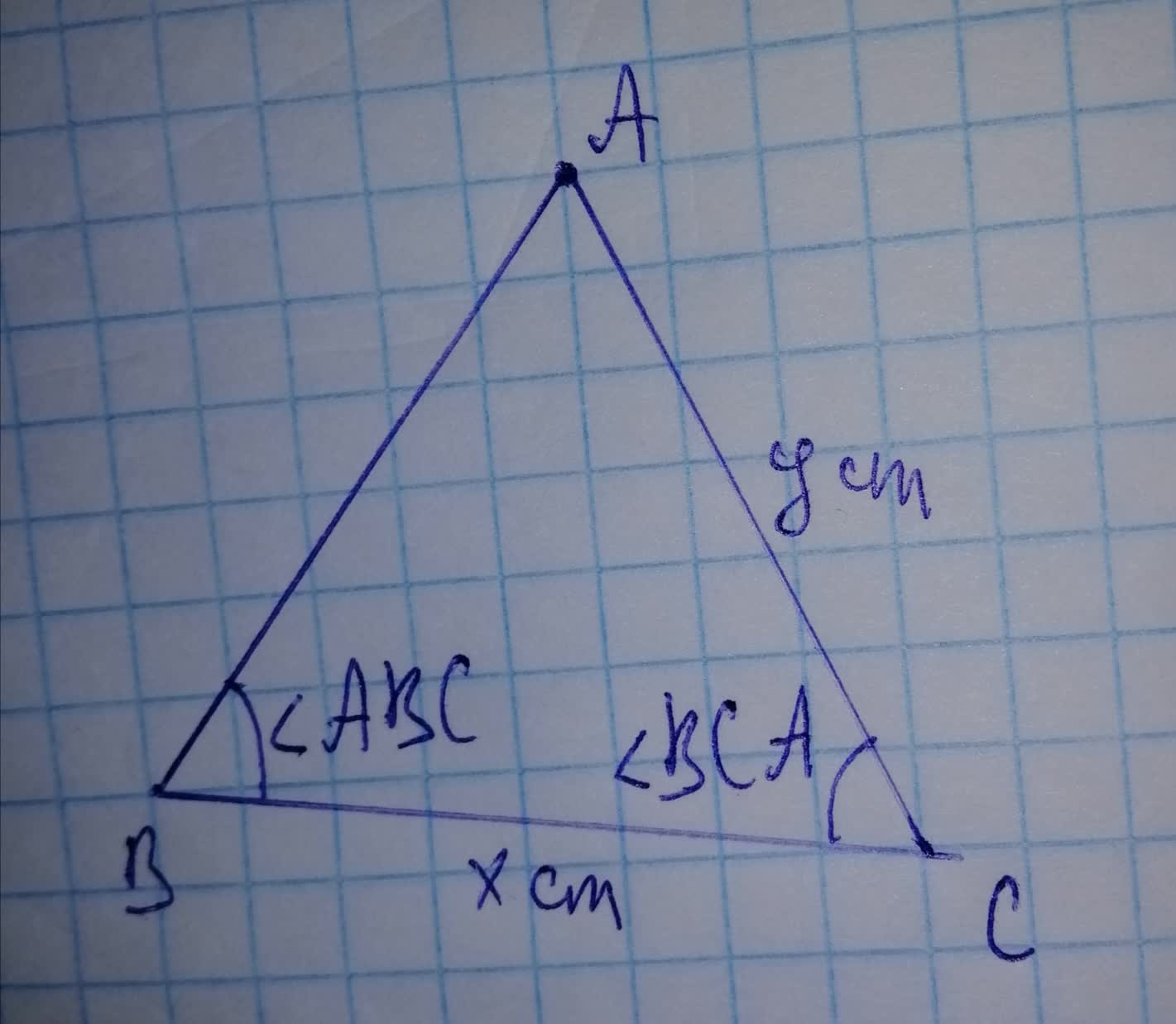# Consider the non-right triangle below. 19610800111.jpg Suppose that m\angle BCA=70^{\circ}, and thatmeplasemamiuk 2021-11-22 Answered
Consider the non-right triangle below.Suppose that $m\mathrm{\angle }BCA={70}^{\circ }$, and that $x=33cm$ and $y=47cm$ What is the degree measure of $\mathrm{\angle }ABC?$
You can still ask an expert for help

• Questions are typically answered in as fast as 30 minutes

Solve your problem for the price of one coffee

• Math expert for every subject
• Pay only if we can solve itGlenn Cooper
Step 1
Using the cosine law,
$AB=\sqrt{A{C}^{2}+B{C}^{2}-\left(2\cdot AC\cdot BC\mathrm{cos}\mathrm{\angle }BCA\right)}$
$AB=\sqrt{{47}^{2}+{33}^{2}-\left(2\cdot 47\cdot 33{\mathrm{cos}70}^{\circ }\right)}$
$AB=\sqrt{2237.05}$
$AB=47.29$
Step 2
Using the cosine law,
$\mathrm{\angle }ABC={\mathrm{cos}}^{-1}\left(\frac{B{C}^{2}+A{B}^{2}-A{C}^{2}}{2×BC×AB}\right)$
$\mathrm{\angle }ABC={\mathrm{cos}}^{-1}\left(0.3576\right)$
$\mathrm{\angle }ABC={69.04}^{\circ }$